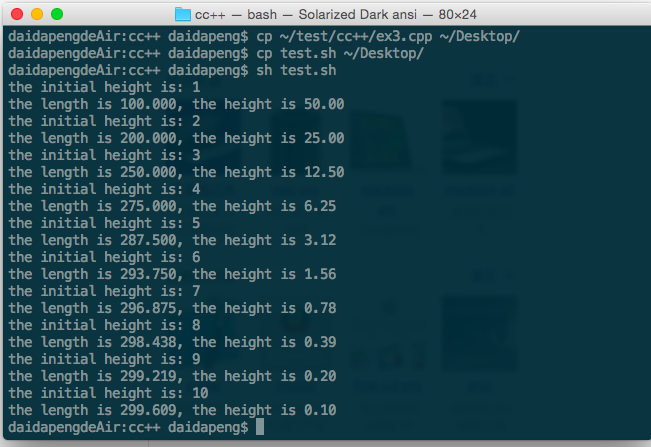# 编写自动测试c语言程序的shell脚本

［一球从 100米高度自由落下,每次落地后反跳回原高度的一半;再落下,求它在10 次落地时,共经过多少米?第10 次反弹多高? ］

#include <stdio.h>
#include <stdlib.h>
#define H 100

int main(int argc, char* argv[])
{
float h0=H;
float sum=H;
float h=h0;
int count=2;
int Number=atoi(argv);
for(;count<=Number;count++)
{

h=h/2.0;
sum+=2*h;
}
h=h/2.0;
printf("the initial height is: %d\n", Number);
printf("the length is %.3f, the height is %.2f\n", sum, h);
return 0;
}


#!/bin/bash

for((i=1;i<=10;i=i+1))
do
./a.out \$i
donefile:///Users/daidapeng/Desktop/屏幕快照%202015-07-10%20下午11.38.01.png